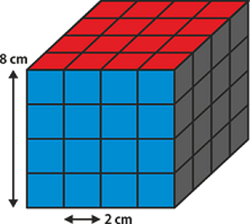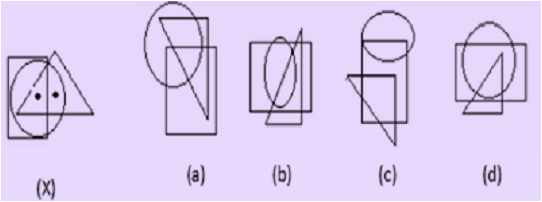# Unified Council - UIMO PDF Sample Papers for Class 8

Class 8 sample paper & practice questions for Unified International Mathematics Olympiad (UIMO) level 1 are given below. Syllabus for level 1 is also mentioned for these exams. You can refer these sample paper & quiz for preparing for the UIMO exam.#### Resources:

##### Sample Questions from Olympiad Success:
 Q.1 Q.2 Q.3 Q.4 Q.5 Q.6 Q.7 Q.8 Q.9 Q.10
 Q.1 The solution of which of the following equations is a fraction not an integer? a) 3x + 2 = 5x + 2 b) 4x - 18 = 2 c) 4x - 7 = x + 2 d) 5x - 9 = x + 4
 Q.2 Which is the smallest square number that is divisible by each of the number 4, 9 and10? a) 900 b) 810 c) 800 d) 920
 Q.3 Expansion of (x + 3y)(x - y) gives: a) x² + 2xy - 3y² b) x + 3y - 2xy c) 2y + 3x d) 3y - 42x
 Q.4 A solid cube of each side 8 cm, has been painted red, blue and black on pairs of opposite faces. It is then cut into cubical blocks of each side 2 cm. How many cubes have only two faces painted?a) 24 b) 2 c) 22 d) 12
 Q.5 What is the mean of all the odd numbers greater than 10 and less than 30? a) 18.5 b) 19 c) 19.5 d) 20
 Q.6 Which of the following is a factor of xy + x - y -1? a) x + 1 b) y + 1 c) x + y d) x - y
 Q.7 Factorise 49x² – 36. a) (7x + 6) (7x - 6) b) (7x + 6) 7x - 6 c) 7x + 6 X 7x - 6 d) None of these
 Q.8 The length of the arc of a sector of a circle is 17 cm and the area of the sector is 136 cm2. Calculate the radius of the circle. a) 16 cm b) 15 cm c) 14 cm d) 13 cm
 Q.9 Select the figure which satisfies the same conditions of placement of the dots as in fig. (X).a) a b) b c) c d) d
 Q.10 Simple interest on a certain sum is 16/25 of the sum. Find the rate percent and the time if both are numerically equal. a) 64% and 64 b) 8% and 8 c) 12% and 12 d) 8.2% and 8.2Sample PDF of Unified Council - Unified International Mathematics Olympiad (UIMO) PDF Sample Papers for Class 8:

 Q.1 )d Q.2 )a Q.3 )a Q.4 )a Q.5 )d Q.6 )b Q.7 )a Q.8 )a Q.9 )a Q.10 )b

Q.1 : d | Q.2 : a | Q.3 : a | Q.4 : a | Q.5 : d | Q.6 : b | Q.7 : a | Q.8 : a | Q.9 : a | Q.10 : b